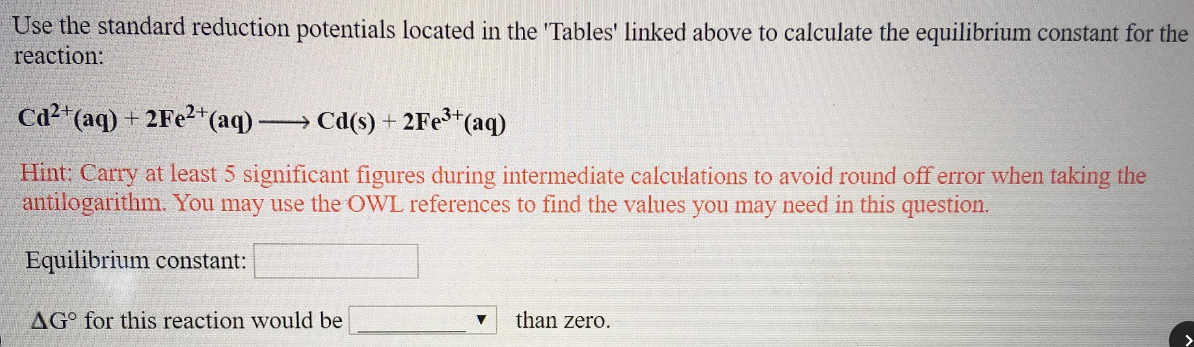# Use the standard reduction potentials located in the 'tables' linked above to calculate the equilibrium constant for the reaction: Cd^2+(aq) + 2Fe^2+(aq) → Cd(s) + 2Fe^3+(aq) Hint: Carry at least 5 significant figures during intermediate calculations to avoid round off error when taking the antilogarithm. you may use the OWL references to find the values you may need in this question. Equilibrium constant: ____ ΔG° for this reaction would be ____ than zero.